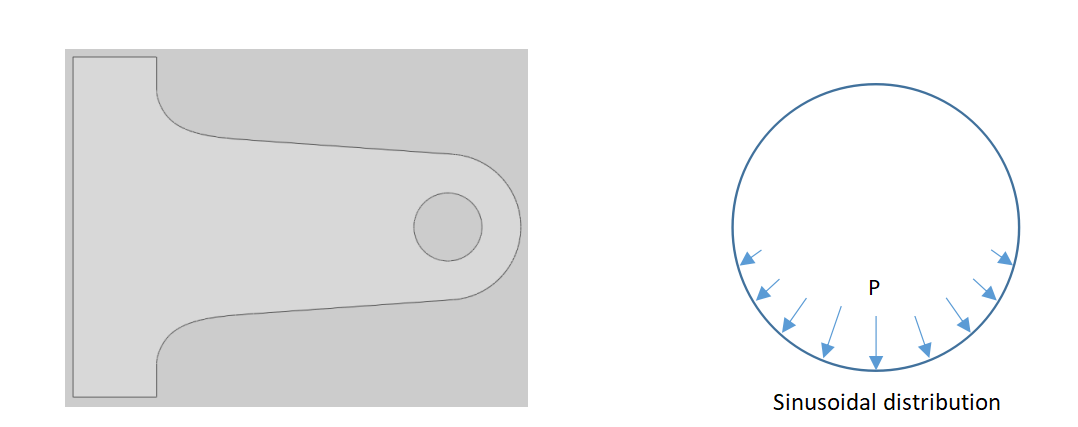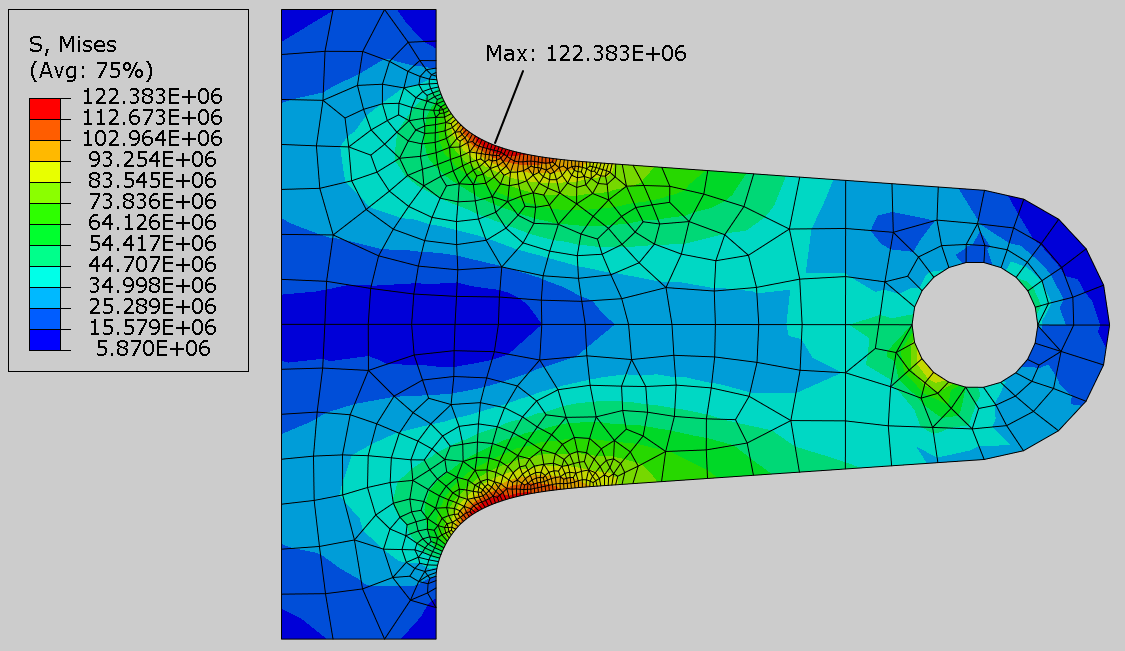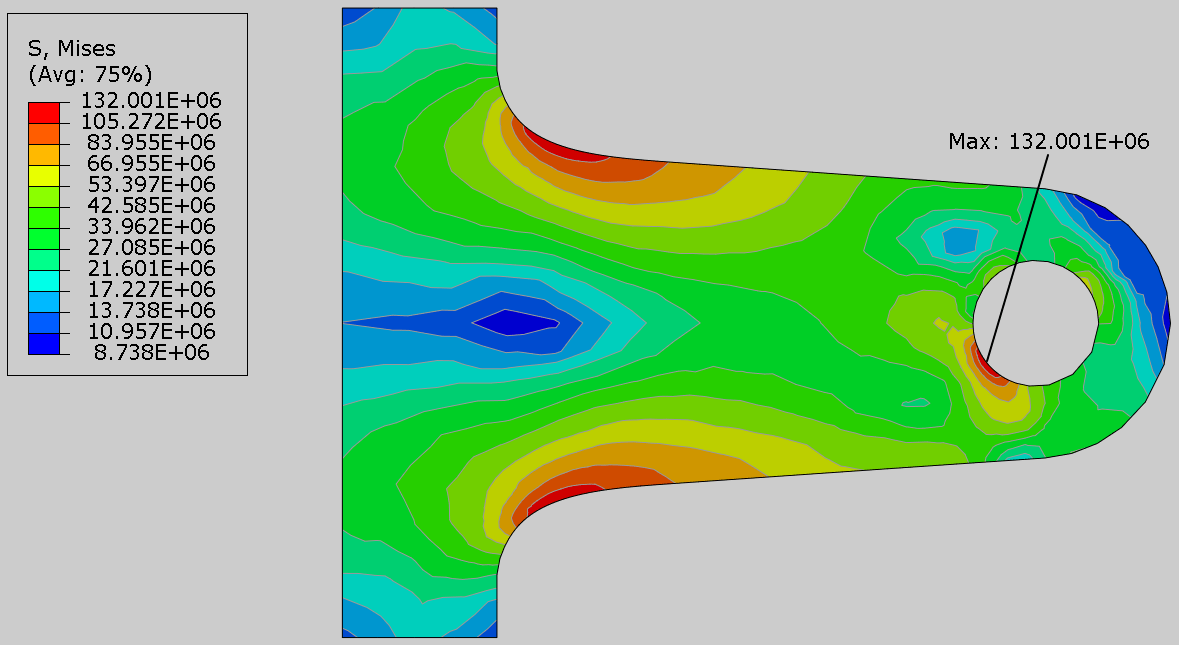## Blog

### Why adaptive remeshing is a must for an efficient and accurate FE solution

Suppose that you need to perform a finite element analysis of the bracket shown in Figure 1. The bracket is fixed on the left side. It is assumed that load is transferred to the bracket by a contacting pin inside the hole. It results in a non-uniform distribution of contacting pressure. We assume that contacting pressure can be represented by a sinusoidally distributed pressure load to simplify the problem. The load is applied slowly such that inertia effects can be neglected. So analysis can be performed using the static procedure.Figure 1: Bracket geometry and sinusoidal distribution of pressure in the hole

The goal of the analysis is to find out the maximum von Mises stress in the part. For that purpose one would like to know the right mesh density. In other words one needs to perform a convergence study. There are three main strategies which are used in practice for mesh refinement. One strategy is to refine mesh globally i.e. throughout the modeling domain. It is very convenient to control the global mesh size and requires little effort on the behalf of an analyst. The drawback with this strategy is that it results in models with large degrees of freedom as this strategy results in finer meshes even in the regions where coarser mesh is sufficient. The Figure 2 shows the mesh generation for the bracket using global mesh refinement strategy with two different mesh sizes.Figure 2: Mesh refinement using global mesh size with two different mesh sizes

Second strategy is to perform local mesh refinement in the regions where high stress gradients occur. To find out such regions, an initial analysis is performed using a coarse mesh. The results for the initial analysis are shown in the Figure 3.Figure 3: Contour plot of von Mises stress for coarse mesh

Th Figure 3 shows that the highest stress of 104.479 MPa occurs close to the radius when coarse mesh is used. So we can expect that highest stress will occur in this region when the mesh will be refined. The expectation seem reasonable even if one makes rough estimation using hand calculations. To find out accurate value of the highest stress we need to refine mesh along the radius. The results for FE analysis using locally refined mesh along the radiuses is shown in the Figure 4. A highest stress of 122.383 MPa is predicted in this region.Figure 4: Contour plot of von Mises stress for locally refined mesh

The mesh seems quite refined along the radiuses so we can say that the maximum stress value in this analysis is our value of interest. But before making a conclusion, one needs to pause for a moment and contemplate if there is a better way to know where the mesh needs to be refined? Fortunately, there is a better way and more surprisingly it is all automatic, using the power of computer. It is called adaptive mesh refinement and available in many FEA software.  Unfortunately most of the analysts in the beginning of their careers, are not aware of the adaptive remeshing which can improve the quality of the solution significantly.

Adaptive remeshing generates a new mesh such as to refine/coarsen mesh depending upon the extent and distribution of discretization error. The discretization error is computed by performing an initial analysis. The error is estimated based on the assumption that the solution should be continuous over the elements and that jumps in the stresses are an indication of discretization error. The initial analysis results contain the information about the distribution of error. In the next step, element sizes are computed based on the distribution of error estimator. Generally, the element sizes are reduced in the region of greatest error estimator and increased in the region of the lowest error estimator. In the next step a new mesh is generated according to the computed element sizes.  Another analysis is performed by considering this new mesh. The results of this analysis are used to judge if mesh refinement is sufficient or further refinement is needed. This process can take many iterations but usually three iterations are sufficient if initial mesh is not too coarse.

The Figure 5 shows the analysis results for the adaptive remeshing process after three iterations. The element edges are not shown for better view. Surprisingly highest stress of 132.001 MPa occurs close to the hole. This value is considerably higher than the maximum value of stress near the radiuses. This highlights how the local mesh refinement strategy could lead us to a wrong conclusion for even such a simple geometry.Figure 5: Contour plot of von Mises stress using adaptive mesh refinement at the end of third iteration

The Figure 6 shows the part geometry with elements generated using adaptive meshing used in the analysis during third iteration. It can be seen that some areas have very high mesh density and others have coarser mesh. Without adaptive remeshing it is very laborious job to locally refine the mesh in specific areas and even more challenging is to know in which areas mesh should be refined. The process of adaptive meshing is automated and software does all of the mesh refinement in the regions where it is required. Adaptive meshing is a great tool for accuracy control. It can significantly improve the quality of simulation results. The goal of adaptive mesh refinement is to obtain a solution that improves the accuracy of finite element solution, while minimizing the number of elements and, hence, the cost of analysis.Figure 6: Mesh generated using adaptive mesh refinement

In the Figure 7, convergence of stresses near the hole and the radius is shown when adaptive meshing is used. The mesh converges quickly without adding too many degrees of freedom (DOF) as the refinement is performed only in the regions where it is required. The number of degrees of freedom is related to the number of nodes. As more elements are added to the modeling domain, the number of degrees of freedom also increases. It is a frequently used metric for reporting convergence studies.Figure 7: Convergence of stresses using adaptive mesh refinement

The Figure 8 shows the convergence of stresses when the bracket is meshed using global meshing strategy. It can be seen that degrees of freedom increase exponentially when this strategy is used.Figure 8: Convergence of stresses using global mesh refinement

By comparing the convergence plots, it can be said that rate of mesh convergence is much faster when adaptive mesh refinement is used.

Concluding remarks

Although global meshing strategy is very convenient to refine mesh, it results in large degrees of freedom. It requires longer computation times though time required to setup model is minimal. In contrast, local mesh refinement strategy results in models with fewer degrees of freedom but requires some insight of the problem. It usually requires considerable effort to setup model e.g. to partition and specifying individual mesh sizes for each region. With this strategy, it is not unusual to overlook a region of interest e.g. a region with potentially a high value of stress. Adaptive meshing performs automatic mesh refinement by first computing discretization error and then generating new mesh to reduce the error. This results in very efficient process and usually after two or three iterations, a mesh is generated which gives accurate solution.

If you want to learn adaptive meshing in depth, you could have a look at the online course “Solving Non-linear Problems with Abaqus